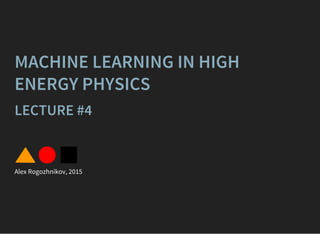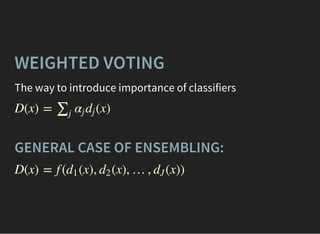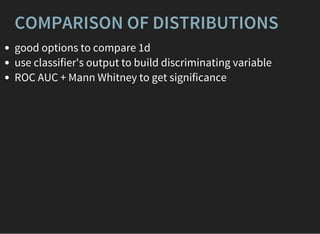Diese Präsentation wurde erfolgreich gemeldet.

# MLHEP 2015: Introductory Lecture #4

Anzeige
Anzeige
Anzeige
Anzeige
Anzeige
Anzeige
Anzeige
Anzeige
Anzeige
Anzeige
Anzeige×

1 von 64 Anzeige

# MLHEP 2015: Introductory Lecture #4

* tuning gradient boosting over decision trees (GBDT)
* speeding up predictions for online triggers: lookup tables
* PCA, autoencoder, manifold learning
* structural learning: Markov chain, LDA
* remarks on collaborative research

* tuning gradient boosting over decision trees (GBDT)
* speeding up predictions for online triggers: lookup tables
* PCA, autoencoder, manifold learning
* structural learning: Markov chain, LDA
* remarks on collaborative research

Anzeige
Anzeige

### MLHEP 2015: Introductory Lecture #4

1. 1. MACHINE LEARNING IN HIGH ENERGY PHYSICS LECTURE #4 Alex Rogozhnikov, 2015
2. 2. WEIGHTED VOTING The way to introduce importance of classifiers D(x) = (x)∑j αj dj GENERAL CASE OF ENSEMBLING: D(x) = f ( (x), (x), …, (x))d1 d2 dJ
3. 3. COMPARISON OF DISTRIBUTIONS good options to compare 1d use classifier's output to build discriminating variable ROC AUC + Mann Whitney to get significance
4. 4. RANDOM FOREST composition of independent trees
5. 5. ADABOOST After building th base classifier:j 1. 2. increase weight of misclassified = ln ( ) αj 1 2 wcorrect wwrong ← ×wi wi e − ( )αj yi dj xi
6. 6. GRADIENT BOOSTING  → min (x) = (x)Dj ∑j =1j ′ αj ′ dj ′ (x) = (x) + (x)Dj Dj−1 αj dj At th iteration:j pseudo-residual train regressor to minimize MSE: find optimal = − zi ∂ ∂D( )xi ∣∣D(x)= (x)Dj−1 dj ( ( ) − → min∑i dj xi zi ) 2 αj
7. 7. LOSSES regression, Mean Squared Error Mean Absolute Error binary classification, ExpLoss (aka AdaLoss) LogLoss ranking losses boosting to uniformity: FlatnessLoss y ∈ ℝ (d( ) −∑i xi yi ) 2 d( ) −∑i ∣∣ xi yi ∣∣ = ±1yi ∑i e − d( )yi xi log(1 + )∑i e − d( )yi xi
8. 8. TUNING GRADIENT BOOSTING OVER DECISION TREES Parameters: loss function pre-pruning: maximal depth, minimal leaf size subsample, max_features = (learning_rate) number of trees N/3 η
9. 9. LOSS FUNCTION different combinations also may be used
10. 10. TUNING GBDT 1. set high, but feasible number of trees 2. find optimal parameters by checking combinations 3. decrease learning rate, increase number of trees See also: GBDT tutorial
11. 11. BaggingClassifier(base_estimator=GradientBoostingClassifier(), n_estimators=100) ENSEMBLES: BAGGING OVER BOOSTING Different variations , are claimed to overcome single GBDT.   Very complex training, better quality if GB estimators are overfitted
12. 12. ENSEMBLES: STACKING Correcting output of several classifiers with new classifier. D(x) = f ( (x), (x), …, (x))d1 d2 dJ To use unbiased predictions, use holdout or kFolding.
13. 13. REWEIGHTING Given two distributions: target and original, find new weights for original distributions, that distributions will coincide.
14. 14. REWEIGHTING Solution for 1 or 2 variables: reweight using bins (so-called 'histogram division'). Typical application: Monte-Carlo reweighting.
15. 15. REWEIGHTING WITH BINS Splitting all space in bins, for each bin: compute , in each bin multiply weights of original distribution in bin to compensate the difference woriginal wtarget ←wi wi wtarget woriginal Problems good in 1d, works sometimes in 2d, nearly impossible in dimensions > 2 too few events in bin reweighting rule is unstable we can reweight several times over 1d if variables aren't correlated ⇒
16. 16. reweighting using enhanced version of bin reweighter
17. 17. reweighting using gradient boosted reweighter
18. 18. GRADIENT BOOSTED REWEIGHTER works well in high dimensions works with correlated variables produces more stable reweighting rule
19. 19. HOW DOES GB REWEIGHTER WORKS? iteratively: 1. Find the tree, which is able to discriminate two distributions 2. Correct weights in each leaf 3. Reweight original distribution and repeat the process. Less bins, bins are guaranteed to have high statistic in each.
20. 20. DISCRIMINATING TREE When looking for a tree with good discrimination, optimize =χ2 ∑l∈leaves ( −wl,target wl,original ) 2 +wl,target wl,original As before, using greedy minimization to build a tree, which provides maximal .χ2 + introducing all heuristics from standard GB.
21. 21. QUALITY ESTIMATION Quality of model can be estimated by the value of optimized loss function. Though remember that different classifiers use different optimization target LogLoss ExpLoss
22. 22. QUALITY ESTIMATION different target of optimization use general quality metrics ⇒
23. 23. TESTING HYPOTHESIS WITH CUT Example: we test hypothesis that there is signal channel with fixed vs. no signal channel ( ).Br ≠ 0 Br = 0 : signal channel with : there is no signal channel H0 Br = const H1 Putting a threshold: d(x) > threshold Estimate number of signal / bkg events that will be selected: s = α tpr, b = β fpr : : H0 ∼ Poiss(s + b)nobs H1 ∼ Poiss(b)nobs
24. 24. TESTING HYPOTHESIS WITH CUT Select some appropriate metric: = 2(s + b) log(1 + ) − 2sAMS 2 s b Maximize it by selecting best special holdout shall be used or use kFolding very poor usage of information from classifier threshold
25. 25. TESTING HYPOTHESIS WITH BINS Splitting all data in bins: . n x ∈ k-th bin ⇔ < d(x) ≤thrk−1 thrk Expected amounts of signal: = α( − ), = β( − )sk tpr k−1 tpr k bk fpr k−1 fpr k : : H0 ∼ Poiss( + )nk sk bk H1 ∼ Poiss( )nk bk Optimal statistics: , = log(1 + ) ∑ k ck nk ck sk bk take some approximation to test power and optimize thresholds
26. 26. FINDING OPTIMAL PARAMETERS some algorithms have many parameters not all the parameters are guessed checking all combinations takes too long
27. 27. FINDING OPTIMAL PARAMETERS randomly picking parameters is a partial solution given a target optimal value we can optimize it no gradient with respect to parameters noisy results function reconstruction is a problem Before running grid optimization make sure your metric is stable (i.e. by train/testing on different subsets). Overfitting by using many attempts is real issue.
28. 28. OPTIMAL GRID SEARCH stochastic optimization (Metropolis-Hastings, annealing) regression techniques, reusing all known information (ML to optimize ML!)
29. 29. OPTIMAL GRID SEARCH USING REGRESSION General algorithm (point of grid = set of parameters): 1. evaluations at random points 2. build regression model based on known results 3. select the point with best expected quality according to trained model 4. evaluate quality at this points 5. Go to 2 if not enough evaluations Why not using linear regression?
30. 30. GAUSSIAN PROCESSES FOR REGRESSION Some definitions: , where and are functions of mean and covariance: , Y ∼ GP(m, K) m K m(x) K( , )x1 x2 represents our prior expectation of quality (may taken constant) represents influence of known results on expectation of new RBF kernel is the most useful: m(x) = Y(x) K( , ) = Y( )Y( )x1 x2 x1 x2 K( , ) = exp(−| − )x1 x2 x1 x2 | 2 We can model the posterior distribution of results in each
31. 31. point. Gaussian Process Demo on Mathematica Also see: .http://www.tmpl.fi/gp/
32. 32. MINUTES BREAKx
33. 33. PREDICTION SPEED Cuts vs classifier cuts are interpretable cuts are applied really fast ML classifiers are applied much slower
34. 34. SPEED UP WAYS Method-specific Logistic regression: sparsity Neural networks: removing neurons GBDT: pruning by selecting best trees Staging of classifiers (a-la triggers)
35. 35. SPEED UP: LOOKUP TABLES Split space of features into bins, simple piecewise-constant function
36. 36. SPEED UP: LOOKUP TABLES Training: 1. split each variable into bins 2. replace values with index of bin 3. train any classifier on indices of bins 4. create lookup table (evaluate answer for each combination of bins) Prediction: 1. convert features to bins' indices 2. take answer from lookup table
37. 37. LOOKUP TABLES speed is comparable to cuts allows to use any ML model behind used in LHCb topological trigger ('Bonsai BDT') Problems: too many combination when number of features N > 10 (8 bins in 10 features), finding optimal thresholds of bins ∼ 1Gb8 10
38. 38. UNSUPERVISED LEARNING: PCA [PEARSON, 1901] PCA is finding axes along which variation is maximal (based on principal axis theorem)
39. 39. PCA DESCRIPTION PCA is based on the principal axes is orthogonal matrix, is diagonal matrix. Q = ΛUU T U Λ Λ = diag( , , …, ), ≥ ≥ ⋯ ≥λ1 λ2 λn λ1 λ2 λn
40. 40. PCA: EIGENFACES Emotion = α[scared] + β[laughs] + γ[angry]+. . .
41. 41. PCA: CALORIMETER Electron/jet recognition in ATLAS
42. 42. AUTOENCODERS autoencoding: , error hidden layer is smaller y = x | − y| → minŷ
43. 43. CONNECTION TO PCA When optimizing MSE: ( − ∑ i ŷ i xi ) 2 And activation is linear: hi ŷ i = = ∑ j wij xi ∑ aij hi
44. 44. j Optimal solution of optimization problem is PCA. BOTTLENECK There is an informational bottleneck algorithm will eager to pass the most precious information. ⇒
45. 45. MANIFOLD LEARNING TECHNIQUES Preserve neighbourhood/distances.
46. 46. Digits samples from MNIST PCA and autoencoder:
47. 47. PART-OF-SPEECH TAGGING
48. 48. MARKOV CHAIN
49. 49. MARKOV CHAIN IN TEXT
50. 50. TOPIC MODELLING (LDA) bag of words generative model that describe different probabilities fitting by maximizing log-likelihood parameters of this model are useful p(x)
51. 51. COLLABORATIVE RESEARCH problem: different environments problem: data ping-pong solution: dedicated machine (server) for a team restart the research = run script or notebook !ping www.bbc.co.uk
52. 52. REPRODUCIBLE RESEARCH readable code code + description + plots together keep versions + reviews Solution: IPython notebook + github Example notebook EVEN MORE REPRODUCIBILITY? Analysis preservation: VM with all needed stuff. Docker
53. 53. BIRD'S EYE VIEW TO ML: Classification Regression Ranking Dimensionality reduction
54. 54. CLUSTERING
55. 55. DENSITY ESTIMATION
56. 56. REPRESENTATION LEARNING Word2vec finds representations of words.
57. 57. THE END